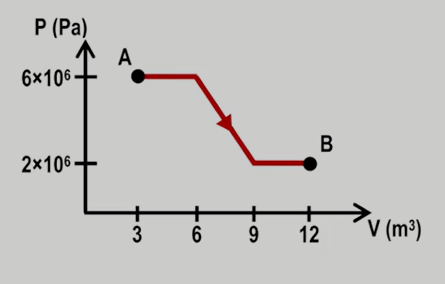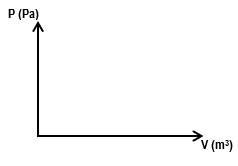Clutch Prep is now a part of Pearson
Ch 23: The First Law of ThermodynamicsWorksheetSee all chapters

# Work Done Through Multiple Processes

See all sections
Sections
Heat Equations for Special Processes & Molar Specific Heats
First Law of Thermodynamics
Work Done Through Multiple Processes
Cyclic Thermodynamic Processes
PV Diagrams & Work

Concept #1: Calculating Works For Multiple Thermodynamic Processes

Practice: How much work is done on a gas that expands from A to B along the path shown below?Practice: A gas with an initial volume of 0.2 m3 is heated at constant volume, and the pressure increases from 2×105 Pa to 5×105. Then, it compresses at constant pressure until it reaches a final volume of 0.12 m3. Draw the two processes in the PV diagram below and find the total work done by the gas.